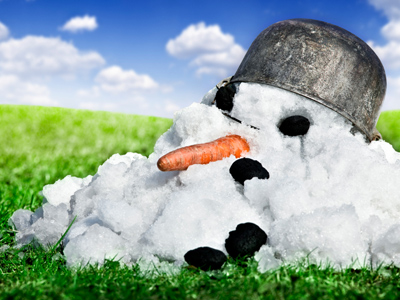The temperature is -13o. If it rises 39o it will be 26o.

# Negative Numbers 2

This Math quiz is called 'Negative Numbers 2' and it has been written by teachers to help you if you are studying the subject at elementary school. Playing educational quizzes is an enjoyable way to learn if you are in the 3rd, 4th or 5th grade - aged 8 to 11.

It costs only \$12.50 per month to play this quiz and over 3,500 others that help you with your school work. You can subscribe on the page at Join Us

Negative or minus numbers are whole numbers below zero. You may wonder where on earth a negative number would be used. An example of how negative numbers are used would be measuring temperatures. If its colder than the freezing point of water (0oC) we say it is a minus temperature. Addition and subtraction can be tricky with negative numbers. One method that might help is to rearrange the problem. For example:

14 - 8 = 6 so 6 - 14 = -8 It all becomes easier with practice!

1.
The temperature is -13o. If it rises 39o what is the temperature now?
26o
39o
13o
-26o
39 - 13 = 26 so -13 + 39 = 26
2.
Which of these statements is true?
-33 > -3
-29 > -28
-7 > -17
-44 > -34
The symbol > means greater than
3.
The temperature is -15o. If it falls 8o what is the temperature now?
23o
-7o
-23o
7o
15 + 8 = 23 so -15 - 8 = -23
4.
The temperature is 6o. If it falls 18o what is the temperature now?
24o
-24o
-8o
-12o
18 - 6 = 12 so 6 - 18 = -12
5.
Which of these statements is true?
-1 < -58
-23 < -1
2 < -99
-15 < -20
The symbol < means smaller than
6.
Which of these is the lowest number?
-1
0
-28
-15
Remember that the higher the negative number the less its value
7.
The temperature is -28o. If it rises 13o what is the temperature now?
-41o
-15o
15o
41o
28 - 13 = 15 so -28 + 13 = -15
8.
Which are the missing numbers in this sequence? -33, -35, ___, -39, -41, ___?
-36 and -43
-37 and -42
-37 and -43
-37 and -40
The numbers in this sequence are decreasing by 2 each time
9.
Which is the largest of these numbers?
2
-35
-3
-14
Even the lowest positive number is higher than all the negative numbers
10.
Which is the next number in the following sequence? 10, 5, 0, -5
10
-1
-10
-15
The numbers are decreasing by 5
Author:  Amanda Swift